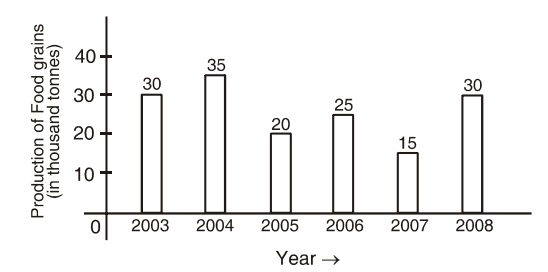Home » Data Interpretation » Bar graph » Question

#### Data Interpretation

Direction: The following bar graph shows the producation of food grains in India during certain year. Study the graph carefully and answer the questions given below:1. Rate of change in quantity of production in which of the following two consecutive years is at the minimum level?
1. 2003 and 2004
2. 2005 and 2006
3. 2007 and 2008
4. 2006 and 2007
##### Correct Option: A

Rate of change in the amount of production in the year 2004 over the year 2003 = (Production in 2004 - Production in 2003 ) x 100/ Production in 2003
Rate of change in the amount of production in the year 2004 over the year 2003 = (35 - 30) x 100 / 30 = 5 x 100 / 30 = 16.67%
Similarly
Rate of change in the amount of production in the year 2006 over the year 2005 = (25 - 20) x 100 / 20 = 5 x 100/20 = 25%
Rate of change in the amount of production in the year 2007 over the year 2008 = (30 - 15) x 100 / 15 = 15 x 100/15= 100%
Rate of change in the amount of production in the year 2006 over the year 2007 = (25 - 15) x 100 / 15 = 10 x 100/15= 66.67%
Hence, it is clear that the rate of change in the amount of production during the consecutive years 2007 and 2008 is at the highest level.